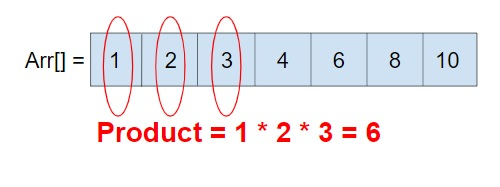# Product of all prime numbers in an Array in C++

Given an integer array arr[] with some elements, the task is to find the product of all the prime number of that numbers.

Prime number are those number which are either divided by 1 or the number itself, or a prime number is a number which is not divisible by any other number except 1 and the number itself. Like 1, 2, 3, 5, 7, 11, etc.

we have to find the solution for the given array −Input −arr[] = { 11, 20, 31, 4, 5, 6, 70 }

Output − 1705

Explanation − Prime numbers in array are − 11, 31, 5 their product is 1705

Input − arr[] = { 1, 2, 3, 4, 5, 6, 7 }

Output − 210

Explanation − Prime numbers in array are − 1, 2, 3, 5, 7 their product is 210

## Approach used below is as follows to solve the problem

• Take input array arr[].

• Loop every element and check if it is prime or not.

• Product all the present primes in an array.

• Return the product.

## Algorithm

Start
In function int prodprimearr(int arr[], int n)
Step 1→ Declare and initialize max_val as max_val *max_element(arr, arr + n)
Step 2→ Declare vector<bool> isprime(max_val + 1, true)
Step 3→ Set isprime and isprime as false
Step 4→ Loop For p = 2 and p * p <= max_val and p++
If isprime[p] == true then,
Loop For i = p * 2 and i <= max_val and i += p
Set isprime[i] as false
Step 5→ Set prod as 1
Step 6→ For i = 0 and i < n and i++
If isprime[arr[i]]
Set prod = prod * arr[i]
Step 6→ Return prod
In function int main(int argc, char const *argv[])
Step 1→ Declare and initilalize arr[] = { 11, 20, 31, 4, 5, 6, 70 }
Step 2→ Declare and initialize n = sizeof(arr) / sizeof(arr)
Step 3→ Print the results of prodprimearr(arr, n)
Stop

## Example

Live Demo

#include <bits/stdc++.h>
using namespace std;
int prodprimearr(int arr[], int n){
// To find the maximum value of an array
int max_val = *max_element(arr, arr + n);
// USE SIEVE TO FIND ALL PRIME NUMBERS LESS
// THAN OR EQUAL TO max_val
vector<bool> isprime(max_val + 1, true);
isprime = false;
isprime = false;
for (int p = 2; p * p <= max_val; p++) {
// If isprime[p] is not changed, then
// it is a prime
if (isprime[p] == true) {
// Update all multiples of p
for (int i = p * 2; i <= max_val; i += p)
isprime[i] = false;
}
}
// Product all primes in arr[]
int prod = 1;
for (int i = 0; i < n; i++)
if (isprime[arr[i]])
prod *= arr[i];
return prod;
}
int main(int argc, char const *argv[]){
int arr[] = { 11, 20, 31, 4, 5, 6, 70 };
int n = sizeof(arr) / sizeof(arr);
cout << prodprimearr(arr, n);
return 0;
}

## Output

If run the above code it will generate the following output −

1705

Updated on: 13-Aug-2020

109 Views